# High School Math : Data Properties

## Example Questions

1 3 Next →

### Example Question #21 : Data Properties

Each student's grade in Becky's math class is determined using a weighted average. Exams comprise 50% of the grade, quizzes comprise 20%, and homework comprises the final 30%. Becky has an 87% average on tests, 82% on quizzes, and 97% on homework. What is her overall grade?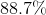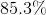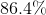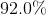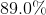Explanation:

First, convert the weighted percents to decimals.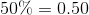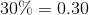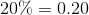Next, multiply each score by the weight.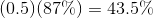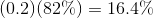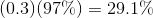Finally, sum all of the weighted scores.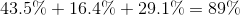### Example Question #22 : Data Properties

Find the mean and median of the following data set: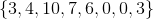Mean:Median: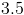Mean:Median:Mean:Median: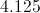Mean:Median:Mean:Median:Mean:Median:Explanation:

The mean is the sum of the numbers divided by their count: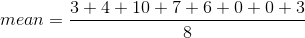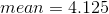To find the median, order the set from smallest to largest: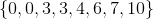When there are two numbers in the middle, find the average of those numbers: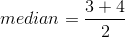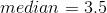### Example Question #23 : Data Properties

Albert's biology grade is based on the average of three test scores and a final.  The final is worth twice a regular test.  What is the minimum grade he must get on the final to get an A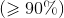in the course if his other test scores were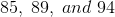?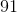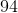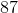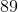Explanation:

The average is the sum of the data divided by the total number of data points.

Let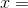final score.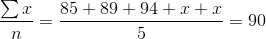Simplify so the equation to solve becomes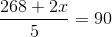.

Cross multiplying we get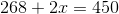.

Then subtract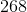from both sides and divide byto get.

1 3 Next →